VS 265

Linear neuron models

Definition of a linear system

• A linear system is one in which the input-output behavior may be described in terms of a linear function.

• What is a linear function? - obeys the rules of superposition and scaling.
• Example: A one-dimensional linear system with input x and output y may be characterized as.

• Linear algebra provides a powerful tool for the analysis of complex, multivariate systems.

• Although most systems in nature are non-linear, our understanding of them can still be aided by the intuitions and insights gained from linear systems analysis.

Neurons
• The brain contains approximatelyneurons. Each neuron can essentially be thought of as a device having a number of inputs and a single output. The inputs consist of the currents generated by the approximately-synapses on the dendritic tree, and the output consists of the action potentials carried by the axon. The axon branches so as to make many copies of the output which are sent on to other neurons.

• The overall input-output characteristic of a neuron is very complicated and still not fully understood. What we do know is that the input currents are generated by ion-specific channels in the membrane which change their conductance in response to chemicals (neurotransmitters) released by other neurons. These currents are (roughly) summed together into the soma, whose voltage rises and decays with the fluctuations in current. When the soma voltage exceeds a certain threshold, an action potential is generated, which then propagates down the axon.

• A conventional way of attempting to understand a neuron is to break it up into many small elements, or compartments. The membrane potential of a given compartment is determined by the conductances of the various ion channels within it. For the Na, K, and Cl ion channels, we havewhereis the membrane voltage,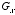denotes the conductance of the ion channel selective to ion x, and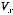denotes the reversal potential (or so-called Nernst potential) of ion x.

• Note thatis not a linear function of the conductances. In addition, the action-potential is a highly non-linear function of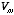.

Linear neurons
• Despite the non-linearities mentioned above, it is still possible to build a simplified, linear model of a neuron that provides useful insights about the function of neurons in the brain.

• A schematic of a linear neuron model is shown below:Each inputis multiplied by a corresponding weight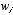and these values are summed together to form the output y. Thus, the output is given as a function of the inputs and weights by the equationor, in vector form,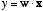, or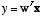.

• The inputsin a linear neuron model can be thought of as the action potentials from other neurons that are impinging upon the neuron’s synapses. The weightscan be thought of as the efficacies of the synapses. The larger, the the moreaffects the neurons output. Some of the factors in a real neuron that would determineare the number of synaptic vescicles in the presynaptic terminal, or the number of ligand-gated channels in the post-synaptic membrane. The sign ofreflects whether it is an excitatory or inhibitory synapse.

• A slight but useful modification of the linear neuron above is to add a non-linear threshold function at the output, which is meant as a crude model of an all-or-nothing action potential generated by a neuron. In this case, the output is given bywhere